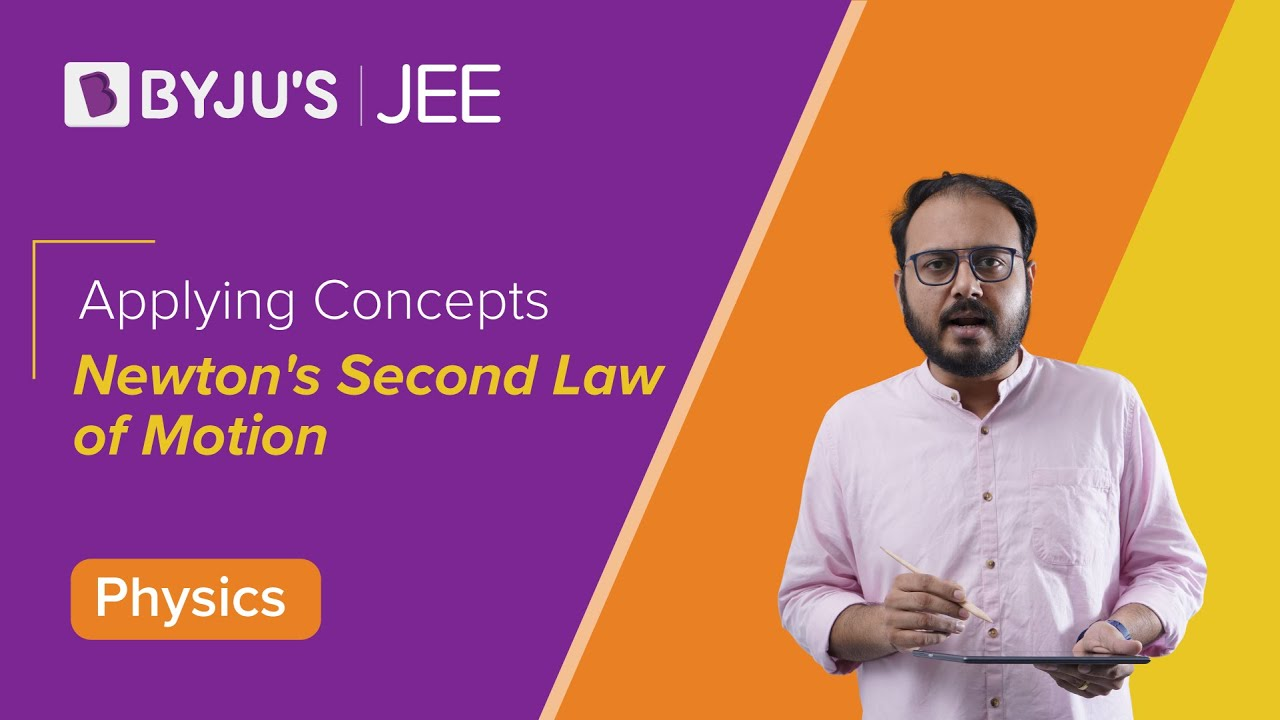Checkout JEE MAINS 2022 Question Paper Analysis : Checkout JEE MAINS 2022 Question Paper Analysis :

# D’Alembert’s Principle

## What is D’Alembert’s Principle?

D’Alembert’s principle states that

For a system of mass of particles, the sum of the difference of the force acting on the system and the time derivatives of the momenta is zero when projected onto any virtual displacement.

It is also known as the Lagrange-d’Alembert principle, named after the French mathematician and physicist Jean le Rond d’Alembert. It is an alternative form of Newton’s second law of motion. According to the 2nd law of motion, F = ma while it is represented as F – ma = 0 in D’Alembert’s law. So it can be said that the object is in equilibrium when a real force is acting on it. Here, F is the real force while -ma is the fictitious force known as inertial force.

## D’Alembert’s Principle Mathematical Representation

D’Alembert’s principle can be explained mathematically in the following way:

$$\begin{array}{l}\sum_{i}(F_{i}-m_{i}a_{i})\delta r_{i}=0\end{array}$$

Where,

• i is the integral used for the identification of variable corresponding to the particular particle in the system
• Fi is the total applied force on the ith place
• mi is the mass of the ith particles
• ai is the acceleration of ith particles
• miai is the time derivative representation
• 𝜹ri is the virtual displacement of ith particle

### Derivation of D’Alembert Principle

Using D’Alembert’s mathematical formula, virtual work can be shown equal to D’Alembert’s principle, which is equal to zero.

Following is the derivation:

$$\begin{array}{l}F_{i}^{(T)} = m_{i}a_{i}\end{array}$$
(total force on each particle)

$$\begin{array}{l}F_{i}^{(T)}-m_{i}a_{i}=0\end{array}$$
(inertial force is moved left, representing quasi-static equilibrium)

$$\begin{array}{l}\delta W = \sum_{i}F_{i}^{(T)}.\delta r_{i}-\sum_{i}m_{i}a_{i}.\delta r_{i}=0\end{array}$$
(equated to virtual work )

$$\begin{array}{l}\delta W = \sum_{i}F_{i}.\delta r_{i}+\sum_{i}C_{i}.\delta r_{i}-\sum_{i}m_{i}a_{i}.\delta r_{i}=0\end{array}$$
(separation of applied force and constraint force)

$$\begin{array}{l}\delta W = \sum_{i}(F_{i}-m_{i}a_{i}).\delta r_{i}=0\end{array}$$
(final equation)

### Examples of D’Alembert Principle

• 1D motion of rigid body: T – W = ma or T = W + ma where T is tension force of wire, W is weight of sample model and ma is acceleration force.
• The 2D motion of rigid body: For an object moving in an x-y plane the following is the mathematical representation: Fi= -mrc where Fi is the total force applied on the ith place, m mass of the body and rc is the position vector of the center of mass of the body.

This is D’Alembert’s principle.

### Applications of D’Alembert’s Principle

D’Alembert’s principle is based on the principle of virtual work along with inertial forces. The following are the applications of D’Alembert’s principle:

• Mass falling under gravity
• Parallel axis theorem
• Frictionless vertical hoop with a bead

Related Physics articles:

## Frequently Asked Questions – FAQs

### What is meant by principle of virtual work?

The principle of virtual work states that when an object is in equilibrium the virtual work done by the forces on the object will be equal to zero. The same is stated in Newton’s laws which state that the applied forces are equal and opposite when at equilibrium.

### What is meant by inertial force?

Inertial force is defined as the force which is in the opposite direction of the accelerating force and is equal to the product of the accelerating force and the mass of the body.

### What is the use of D’Alembert’s principle?

D’Alembert’s principle is used for analyzing the dynamic problem which can reduce it into a static equilibrium problem.

### What is virtual displacement?

A virtual displacement is defined as the instantaneous change in the coordinates of the system.

### What are the quantities related in Newton’s second law of motion?

In Newton’s second law there are two vector quantities, and they are force and acceleration.

## Understanding Newton’s Second Law of MotionStay tuned with BYJU’S for more such interesting articles. Also, register to “BYJU’S – The Learning App” for loads of interactive, engaging Physics-related videos and unlimited academic assistance.

Test your knowledge on Dalemberts Principle